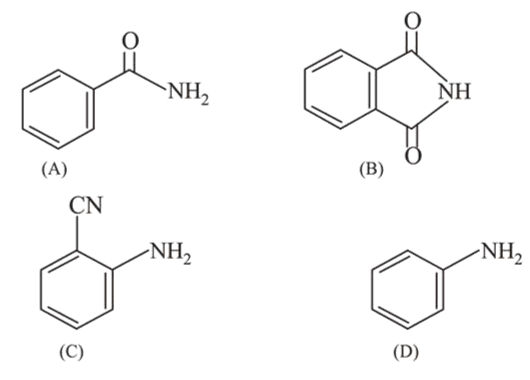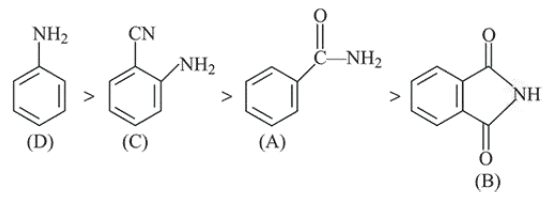# The increasing order of reactivity of the followingQuestion:

The increasing order of reactivity of the following compound towards reaction with alkyl halides directly is:1. $(\mathrm{B})<(\mathrm{A})<(\mathrm{C})<(\mathrm{D})$

2. $(\mathrm{A})<(\mathrm{B})<(\mathrm{C})<(\mathrm{D})$

3. $(B)<(A)<(D)<(C)$

4. $(\mathrm{A})<(\mathrm{C})<(\mathrm{D})<(\mathrm{B})$

Correct Option: 1

Solution: